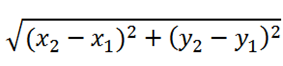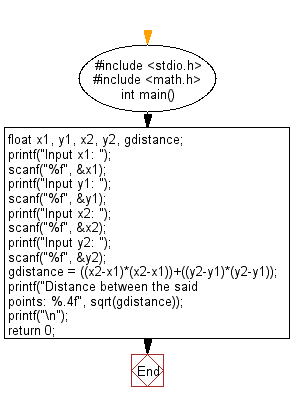﻿ C Program: Calculate the distance between the two points - w3resource

# C Exercises: Calculate the distance between the two points

## C Basic Declarations and Expressions: Exercise-15 with Solution

Write a C program to calculate the distance between two points.
Note: x1, y1, x2, y2 are all double values.

Formula:C Code:

``````#include <stdio.h>
#include <math.h>

int main() {
float x1, y1, x2, y2, gdistance; // Declare variables for coordinates and distance

// Prompt user for coordinates (x1, y1) and store them
printf("Input x1: ");
scanf("%f", &x1);
printf("Input y1: ");
scanf("%f", &y1);

// Prompt user for coordinates (x2, y2) and store them
printf("Input x2: ");
scanf("%f", &x2);
printf("Input y2: ");
scanf("%f", &y2);

// Calculate squared distance between points
gdistance = ((x2-x1)*(x2-x1))+((y2-y1)*(y2-y1));

// Calculate and print the distance between the points
printf("Distance between the said points: %.4f", sqrt(gdistance));
printf("\n");

return 0;
}
``````

Explanation:

In the exercise above -

• First, the program includes two standard libraries: <stdio.h> for input/output functions and <math.h> to use the sqrt() function for calculating the square root.
• It declares the following variables:
• float x1, y1, x2, y2: These variables will store the coordinates of two points in the form (x, y) where (x1, y1) are the coordinates of the first point, and (x2, y2) are the coordinates of the second point.
• float gdistance: This variable stores the squared distance between the two points.
• The program prompts the user to input the coordinates of the first point:
• It uses "printf()" function to display "Input x1:" to instruct the user to input the x-coordinate of the first point.
• It uses "scanf()" function to read the user's input and store it in the variable 'x1'.
• It repeats the same process for the y-coordinate of the first point ('y1') and then for the coordinates of the second point ('x2' and 'y2').
• It calculates the squared distance between the two points using the distance formula for two points (x1, y1) and (x2, y2):
• gdistance = ((x2 - x1)*(x2 - x1)) + ((y2 - y1)*(y2 - y1)): This formula computes the square of the distance between the points.
• The program uses the "printf()" function to display the result:
• It prints "Distance between the said points: " along with the calculated square root of 'gdistance' using sqrt(gdistance).
• The %.4f format specifier displays the result with four decimal places.
• Finally, the program returns 0 to indicate successful execution.

Sample Output:

```Input x1: 25
Input y1: 15
Input x2: 35
Input y2: 10
Distance between the said points: 11.1803
```

Flowchart:C Programming Code Editor:

What is the difficulty level of this exercise?

Test your Programming skills with w3resource's quiz.

﻿